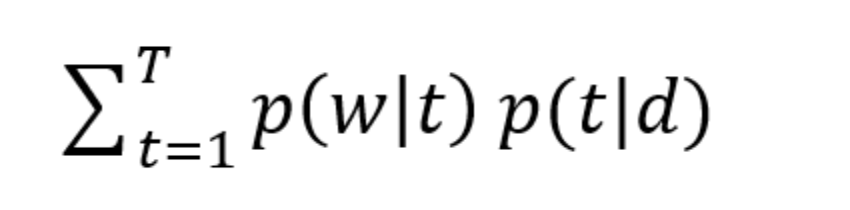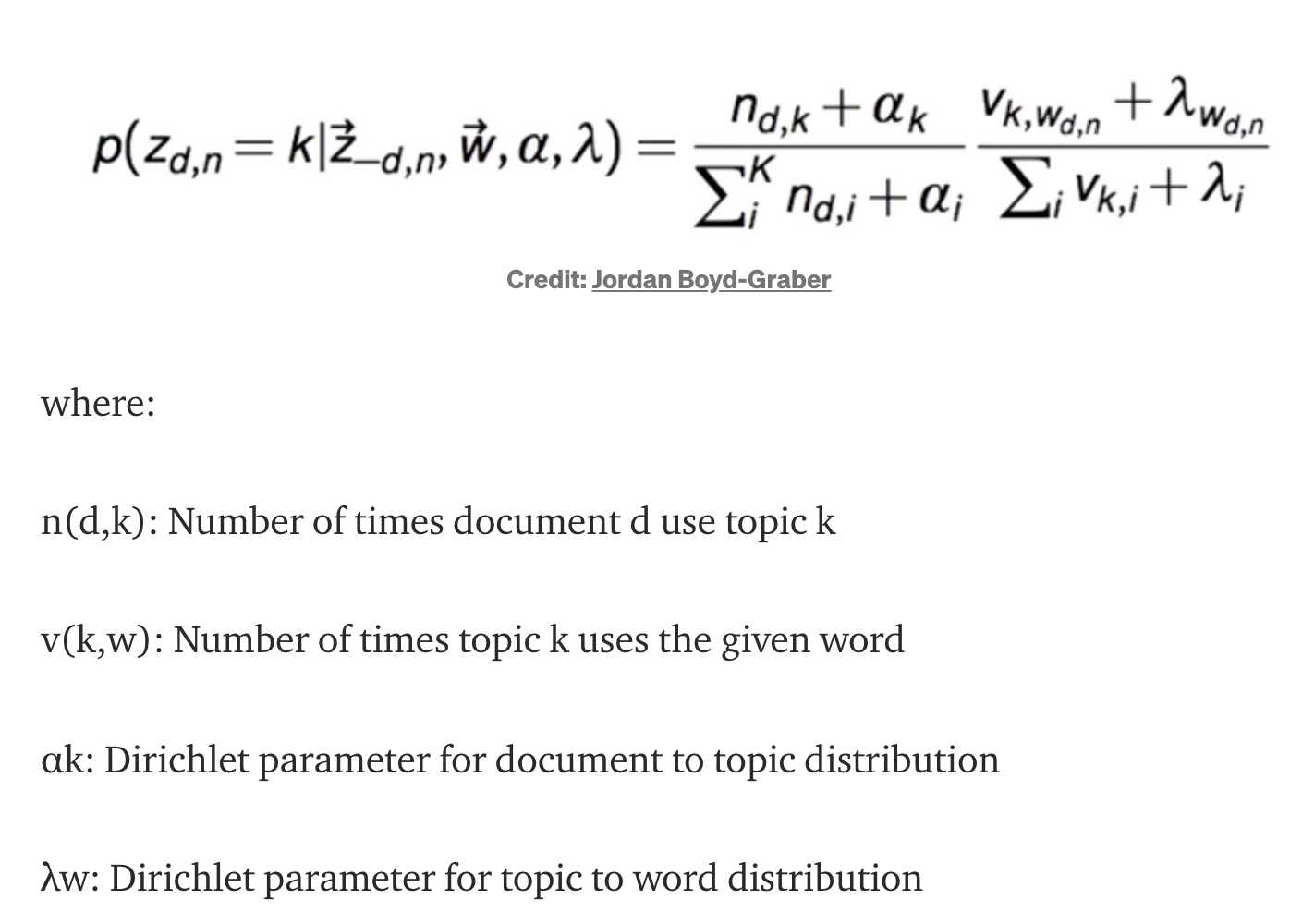Topic Modeling:

Topic modeling is a type of statistical modeling for discovering the abstract “topics” that occur in a collection of documents.

LDA

Latent Dirichlet Allocation (LDA) is an example of topic model and is used to classify text in a document to a particular topic. Modeled as Dirichlet distributions are

• a topic per document model -> a documents consists of multiple words each with a topic distribution
• words per topic model -> every topic is closer to a group of words
In [ ]:
# Eg:
# doc1 = ['Tree has fruits']
# doc2 = ['abc party was elected']


Latent: This refers to everything that we don’t know a priori and are hidden in the data. Here, the themes or topics that document consists of are unknown, but they are believed to be present as the text is generated based on those topics.

Dirichlet: It is a ‘distribution of distributions’.

Allocation: This means that once we have Dirichlet, we will allocate topics to the documents and words of the document to topics.

1. ϴtd = P(t|d) which is the probability distribution of topics in documents
2. Фwt = P(w|t) which is the probability distribution of words in topics And, we can say that the probability of a word given document i.e. P(w|d) is equal to:

### p(w|d) = Σ(p(w|d,t)*p(d/t))¶

sum for all t in T, where T is the total number of topics. Also, let’s assume that there is W number of words in our vocabulary for all the documents. If we assume conditional independence, we can say that P(w|t,d) = P(w|t) And hence P(w|d) is equal to:

that is the dot product of ϴtd and Фwt for each topic t.Gibbs Sampling Gibbs sampling is an algorithm for successively sampling conditional distributions of variables, whose distribution over states converges to the true distribution in the long run. This is somewhat an abstract concept and needs a good understanding of Monte Carlo Markov Chains and Bayes theorem.